• 本文介绍有向图拓扑排序算法的思路及代码实现，首先讲解什么是拓扑排序，其次介绍实现拓扑排序需要的检测有向是否有环的算法及顶点排序算法，最终实现有向拓扑排序。 一、什么是拓扑排序？ 给定一副有向，...
有向图拓扑排序
前言
本文介绍有向图拓扑排序算法的思路及代码实现，首先讲解什么是拓扑排序，其次介绍实现拓扑排序需要的检测有向图是否有环的算法及顶点排序算法，最终实现有向图的拓扑排序。
一、什么是拓扑排序？

给定一副有向图，将所有的顶点排序，使得所有的有向边均从排在前面的元素指向排在后面的元素，此时就可以明
确的表示出每个顶点的优先级。如对下图进行拓扑排序：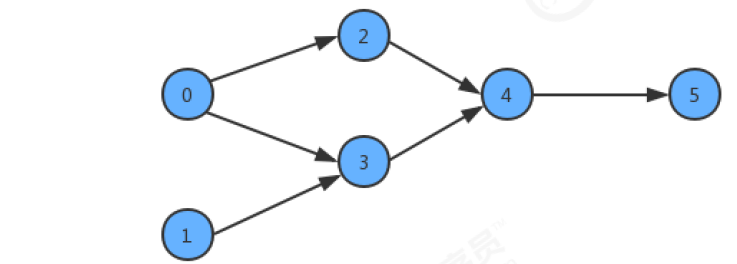拓扑排序结果为：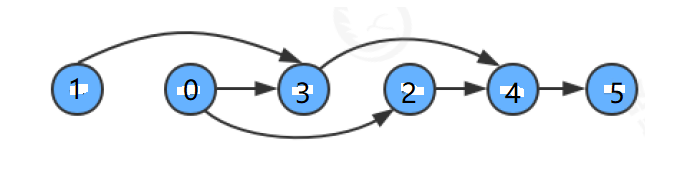根据拓扑排序的概念，如果对有向图进行拓扑排序，那么图中必须没有环，否则，就不能进行拓扑排序，然后在图中无环的情况下，再进行顶点排序，最终实现拓扑排序。

二、检测有向图中是否有环

算法思路：基于深度优先搜索算法检测图中是否有环。1. 定义boolean辅助数组onStack，以栈的思想标识顶点是否在搜索中；2. 在深度优先搜索中，不断检测当前搜索的顶点是否在栈中（即当前顶点的值是否为true，如果为true，则在栈中，否则不在栈中），如果在栈中，说明该顶点被重复搜索到，代表图中有环；3. 每个顶点深度优先搜索完成，onStack需要出栈（即将当前索引对应的值修改为false），为下个顶点搜索做准备
示例：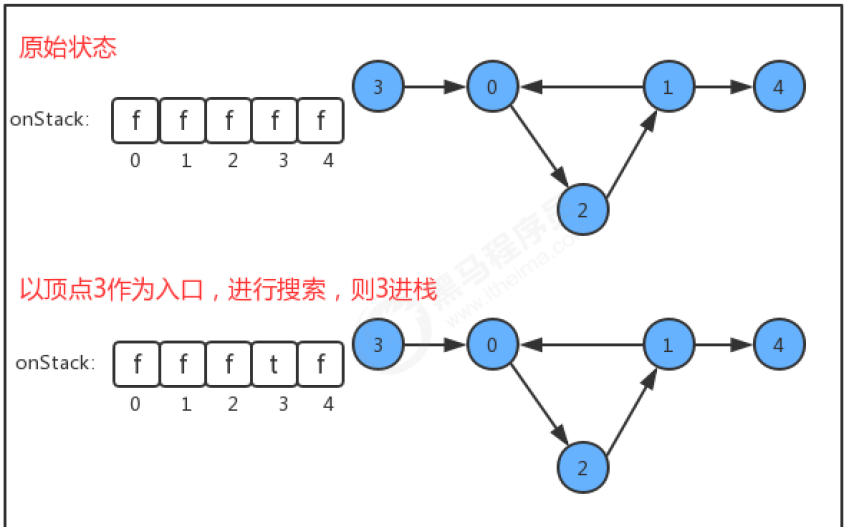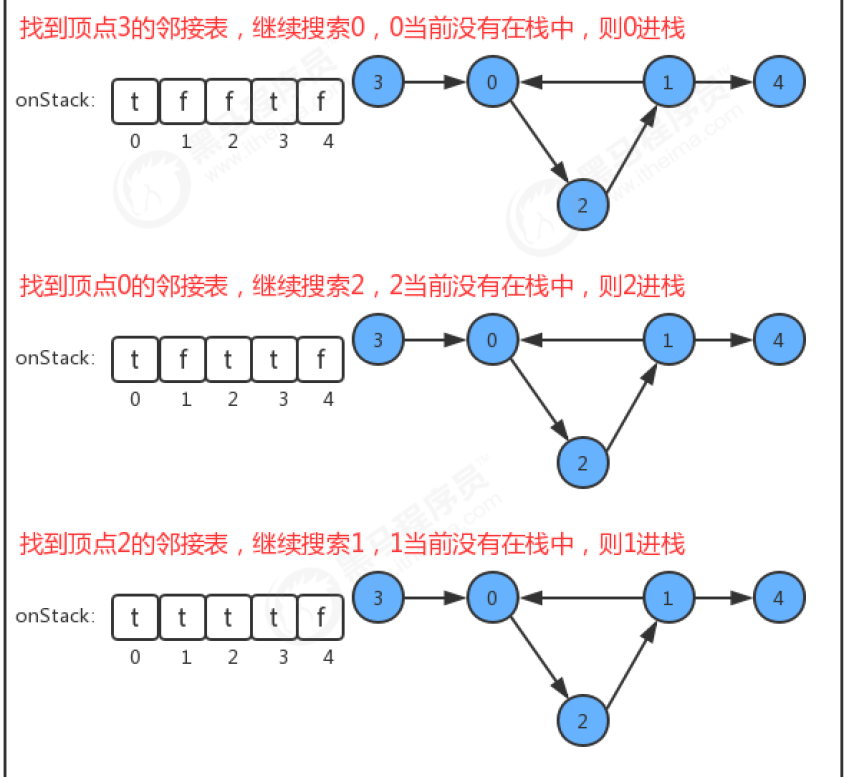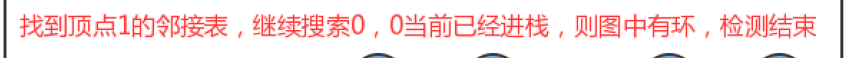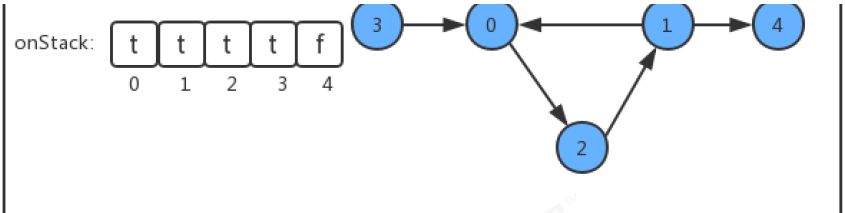代码实现

public class DirectedCycle {
private boolean[] marked;//索引代表顶点，用于标识顶点是否搜索过,是深度优先搜索的复杂数组
private boolean hasCycle;//记录图中是否有环
private boolean[] onStack; //索引代表顶点，使用栈的思想，记录当前顶点有没有已经处于正在搜索的栈上，如果有，则证明有环。

//创建一个检测环对象，检测图G中是否有环
DirectedCycle(DirectGraph G)
{
this.marked=new boolean[G.V()];//用于标识顶点是否搜索过
this.hasCycle=false;
this.onStack=new boolean[G.V()];//用于标识顶点是否在搜索中

//遍历所有顶点，将未搜索过的顶点作为入口，进行深度优先遍历，检测是否有环，一旦检测到有环，则结束；
//因为对于不连通图，有很多个子图，也许某个子图存在环，因此，要对每个子图进行深度优先遍历检测，而不能只检测某一个子图。
for (int v = 0; v < G.V(); v++) {
if (!marked[v])
dfs(G,v);//每次搜索一个子图，判断子图内是否有环，如果没环，继续搜索下一个子图（一次搜索后，未搜索的顶点一定在另一个子图中）
}
}

//基于深度优先搜索，检测图G中是否有环
private void dfs(DirectGraph G,int v)
{
//1.当前顶点标记为已搜索
marked[v]=true;
//2.当前顶点入栈
onStack[v]=true;
//3.递归深度优先遍历，检查遍历结点是否已经在栈中，如果在栈中，则表明该顶点被两次搜索到，证明有环，则结束
for (Integer w : G.adj(v)) {
if (!marked[w])
dfs(G,w);
//如果该顶点已经被搜索过，且如果该顶点在搜索的路径上，则代表又一次搜索到该顶点，证明有环，结束搜索。
if (onStack[w]) {
hasCycle = true;
return;
}
}
//4.当前顶点出栈，为下一个节点作为入口，检测是否有环做准备(为什么需要这样，图2.png可以解释)
onStack[v]=false;
}
//判断当前有向图G中是否有环
public boolean hasCycle()
{
return hasCycle;
}
}

代码中为什么每个顶底深度优先搜索完成后onStack需要出栈，下图可以解释：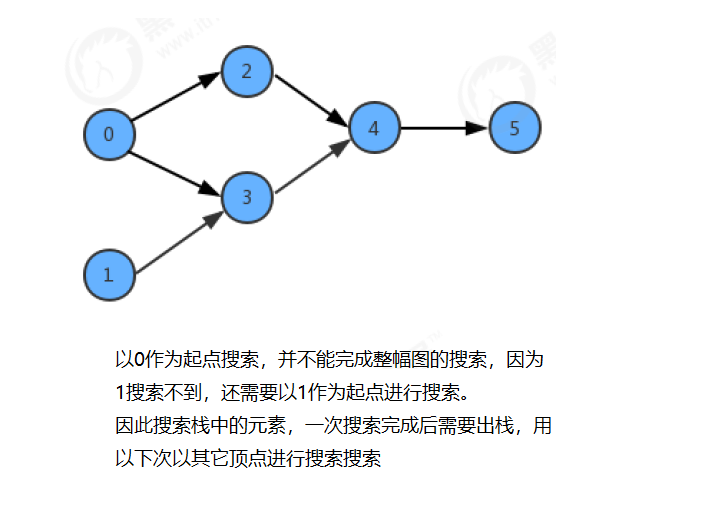三、基于深度优先的顶点排序

拓扑排序使得所有的有向边均从排在前面的元素指向排在后面的元素，要实现这一需要，可以通过顶点排序进行实现。
顶点排序算法思路：1. 定义栈stack用于存储顶点排序的结果；2. 基于深度优先搜索算法，每个顶点深度优先搜索完成后，将该顶点入栈；3. 依次弹出栈中顶点，即为满足拓扑排序要求的顶点序列。
顶点排序示例：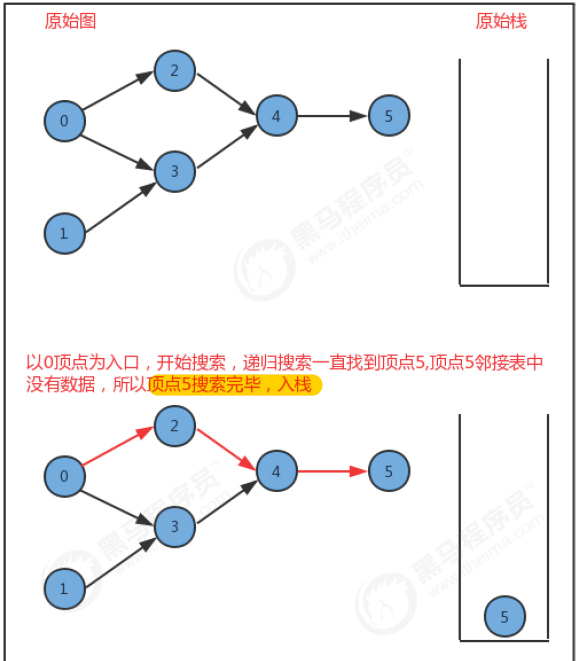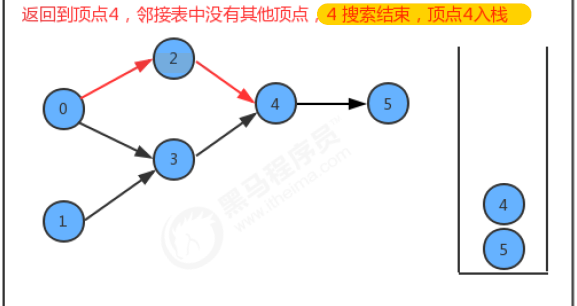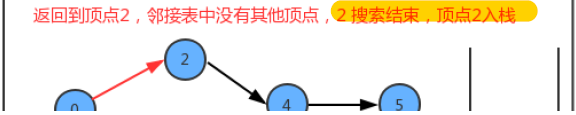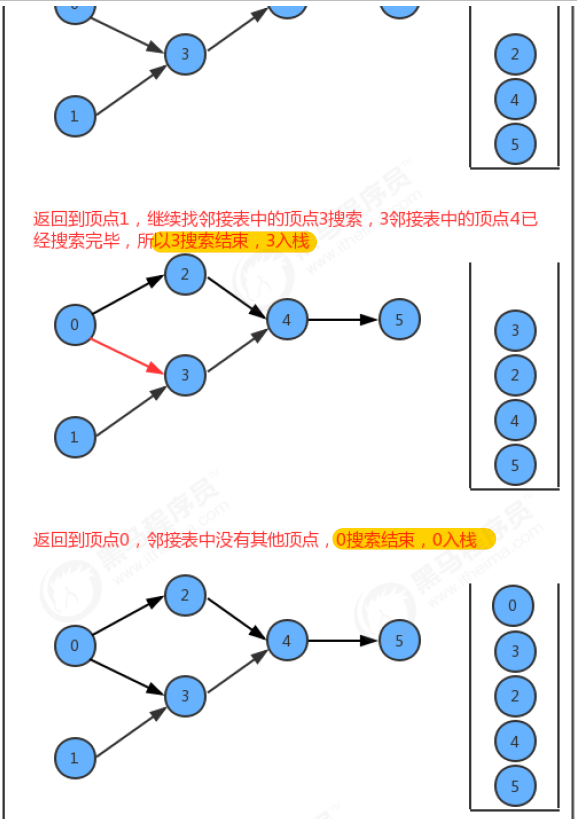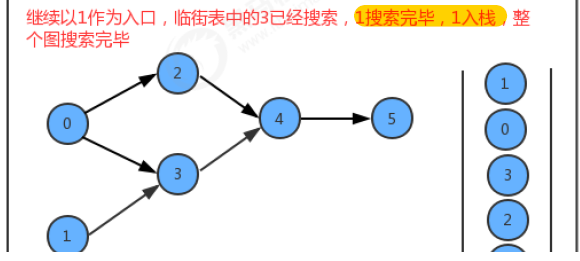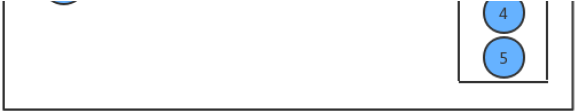代码实现

public class DepthFirstOrder {
private boolean[] marked;//索引代表顶点，值表示当前顶点是否已经被搜索
private Stack<Integer> reversePost;//使用栈，存储顶点序列，打印出栈中的顶点，即是排序后的顶点

public DepthFirstOrder(DirectGraph G)
{
//初始化辅助变量
this.marked=new boolean[G.V()];//默认全部赋值为false
this.reversePost=new Stack<Integer>();
//对每一个未搜索过的顶点进行深度优先遍历
for (int v = 0; v < G.V(); v++) {
if (!marked[v])
dfs(G,v);
}
}

//基于深度优先搜索，生成顶点线性序列
private void dfs(DirectGraph G,int v)
{
//1. 将当前顶点标记为已搜索
marked[v]=true;
//2. 遍历当前顶点的邻接表，对邻接表中未搜索的顶点递归调用深度优先搜索
for (Integer w : G.adj(v)) {
if(!marked[w])
dfs(G,w);
}
//3. 当前顶点v深度优先搜索完毕后，入栈
reversePost.push(v);
}
//获取顶点线性序列
public Stack<Integer> reversePost()
{
return reversePost;
}
}

四、拓扑排序实现

实现了检测是否有环和顶点排序算法，也就完成了拓扑排序，拓扑排序是对上面两个算法的封装。
拓扑排序算法步骤：1. 定义栈用于存储拓扑排序顶底；2. 检测图中是否有环；3. 若有环则不做拓扑排序，若无环则对图进行顶点排序，完成拓扑排序
代码实现

public class TopoLogical {
private Stack<Integer> order; //顶点的拓扑排序

public TopoLogical(DirectGraph G)
{
//1. 检测是否有环
DirectedCycle directedCycle = new DirectedCycle(G);
if (!directedCycle.hasCycle())
{
//2. 调用顶点排序算法
DepthFirstOrder depthFirstOrder = new DepthFirstOrder(G);
this.order=depthFirstOrder.reversePost();
}
}

//判断图G是否有环
public boolean isCycle()
{
return order==null;
}
//获取拓扑排序的所有顶点
public Stack<Integer> order()
{
return order;
}
}



展开全文数据结构
•   有向无环拓扑排序在 https://blog.csdn.net/Bob__yuan/article/details/95613891 也有记录，是通过拓扑排序查看一个是不是无环的。   这篇文章记录的是另一种用法，就是用有向来表示任务的依赖关系，...
  有向无环图的拓扑排序在 https://blog.csdn.net/Bob__yuan/article/details/95613891 也有记录，是通过拓扑排序查看一个图是不是无环的。
这篇文章记录的是另一种用法，就是用有向图来表示任务的依赖关系，然后通过拓扑排序找出按照依赖关系前后顺序完成所有任务的方法。百度里介绍拓扑排序也是说拓扑排序就是这个目的。
就比如说，有 n 个任务需要执行，但是有的任务和任务之间是有依赖关系的，比如穿毛裤之前肯定要先穿秋裤这种感觉。题目是：第一行给出两个正整数 n 和 m，n 表示任务总数，m 表示有 m 个依赖关系，下边一行是 n 个正整数，表示每个任务需要花费的时间（任务从 1 开始计数），接下来 m 行每行两个正整数 xi 、yi 表示 yi 依赖 xi，也就是 xi 必须在 yi 之前完成。如果一个任务必须完成了才能开始新的任务，怎么能让所有任务平均等待时间最短。
肯定要让完成所需时间短的任务先完成，所以需要一个优先队列，还需要考虑的是，先让所有入度 为0（也就是不依赖别的任务）的任务进队列，然后每次有任务完成，如果有任务依赖它，那这个任务的入度就减少1，如果入度减少为 0，说明这个任务前边的依赖任务已经都完成了，就让它入队，代码如下：
#include <iostream>
#include <vector>
#include <queue>
using namespace std;

vector<int> need_time;

struct cmp {
bool operator()(int i, int j) {
if (need_time[i] != need_time[j])
return need_time[i] > need_time[j];
else
return i > j;
}
};

int main() {
int n, m;		// n 个任务，m 个依赖关系
cin >> n >> m;
need_time = vector<int>(n + 1);
vector<int> ins(n + 1, 0);			// 每个节点的入度
vector<pair<int, int>> req(m + 1);	// require
for (int i = 1; i <= n; ++i)
cin >> need_time[i];
for (int i = 1; i <= m; ++i){
cin >> req[i].first >> req[i].second;
++ins[req[i].second];
}

priority_queue<int, vector<int>, cmp> q;

// 入度为0（不依赖别的任务）的任务入队
for (int i = 1; i <= n; ++i)
if(ins[i] == 0)
q.push(i);
while (!q.empty())
{
auto tmp = q.top();
cout << tmp << " ";
q.pop();
for (auto ite = req.begin(); ite != req.end();)	{
if (ite->first == tmp) {
if (--ins[ite->second] == 0)
q.push(ite->second);
ite = req.erase(ite);
}
else
++ite;
}
}
}

也可以把任务依赖关系用一个 unordered_map<int, unordered_set< int >> 来存储，这样每次就不需要遍历了。


展开全文• PAGE 数据结构课程设计 设计说明书 有向图拓扑排序算法的实现 学生姓名 樊佳佳 学号 1318064017 班级 网络工程1301 成绩 指导教师 申静 数学与计算机科学学院 2016年1月4日 课程设计任务书 20152016学年第一学期 ...
• 拓扑排序 拓扑排序是对有向无圈的顶点的一种排序，是得如果存在一条从Vi到Vj的路劲，那么在排序中Vj就出现在Vi的后面。例如课程的学习顺序。
拓扑排序

拓扑排序是对有向无圈图的顶点的一种排序，是得如果存在一条从Vi到Vj的路劲，那么在排序中Vj就出现在Vi的后面。例如课程的学习顺序。

/**
*
* @author 陈鑫
* 图的拓扑排序
*/
public class TopologicalSorting {

static class Vertex{
public String vertexName;//点的名称
public Integer topNum;//拓扑编号
public Integer indegree;//入度

public String getVertexName() {
return vertexName;
}
public void setVertexName(String vertexName) {
this.vertexName = vertexName;
}
public Integer getTopNum() {
}
public void setTopNum(Integer topNum) {
this.topNum = topNum;
}
public Integer getIndegree() {
return indegree;
}
public void setIndegree(Integer indegree) {
this.indegree = indegree;
}
}
}

}
/**
* 拓扑排序
*
*/
int count =0;
//将初始入度为0的顶点入队
if(v.getIndegree() == 0) {
}
}

while(!queueList.isEmpty()) {

Vertex v = queueList.poll();//出队
v.topNum = ++count;//给拓扑编号赋值

/*
* 遍历入度为0顶点的邻接顶点，将入度减1，再找出入度为0的顶点入栈
*/
Integer indegree = w.getIndegree();
w.setIndegree(--indegree);//入度减1
if( indegree == 0) {
}
}
}

}
}

/**
* 计算入度
* @return
*/
public static void getIndegree(ArrayList<Vertex> adjacencyList) {
//初始化
v.setIndegree(0);
}
//计算入度
w.setIndegree(w.getIndegree() + 1);
}
}
}

}

public static void main(String[] args) {
//将图用邻接表表示

Vertex vertex1 = new Vertex();
Vertex vertex2 = new Vertex();
Vertex vertex3 = new Vertex();
Vertex vertex4 = new Vertex();
Vertex vertex5 = new Vertex();
Vertex vertex6 = new Vertex();
Vertex vertex7 = new Vertex();
vertex1.setVertexName("v1");
vertex2.setVertexName("v2");
vertex3.setVertexName("v3");
vertex4.setVertexName("v4");
vertex5.setVertexName("v5");
vertex6.setVertexName("v6");
vertex7.setVertexName("v7");

//计算入度
//拓扑排序
//输出打印
System.out.println(v.getVertexName()+":" + v.getIndegree());//注意：由于排序时入度减一所以入度打印的都是0
}

System.out.println(v.getVertexName()+":" + v.getTopNum());
}

}

}


展开全文数据结构 算法
• （2）拓扑排序不唯一，只需保证各节点在上的原始顺序保持不变； （3）实现（可借助辅助队列或栈，时间复杂度为 O(|E|+|V|)）； import java.util.ArrayList; import java.util.LinkedList; import java.util....
拓扑排序（1）仅针对有向无环图；（2）图的拓扑排序不唯一，只需保证各节点在图上的原始顺序保持不变；（3）实现（可借助辅助队列或栈，时间复杂度为O(|E|+|V|)）；import java.util.ArrayList;
import java.util.List;
import java.util.Queue;

class CycleFoundException extends Exception {}

public class Test {
public static class GrapNode {
public String name;
public int indegree = 0;
public List<GrapNode> neighbors = new ArrayList<GrapNode>();
GrapNode(String name) {
this.name = name;
}
for(GrapNode node : nodes) {
node.indegree++;
}
}
}
public static List<String> topSort(GrapNode... grap) throws CycleFoundException{
List<String> sorted = new ArrayList<String>();
for(GrapNode node : grap) {
if(node.indegree == 0)
}
while(!queue.isEmpty()) {
GrapNode node = queue.remove();
for(GrapNode n : node.neighbors) {
if(--n.indegree == 0)
}
}
if(sorted.size() < grap.length)
throw new CycleFoundException();
return sorted;
}
public static void main(String[] args) {
GrapNode v1 = new GrapNode("v1");
GrapNode v2 = new GrapNode("v2");
GrapNode v3 = new GrapNode("v3");
GrapNode v4 = new GrapNode("v4");
GrapNode v5 = new GrapNode("v5");
GrapNode v6 = new GrapNode("v6");
GrapNode v7 = new GrapNode("v7");
try {
List<String> sorted = topSort(v1,v2,v3,v4,v5,v6,v7);
for(String s : sorted) {
System.out.print(s+", ");
}

} catch (CycleFoundException e) {
e.printStackTrace();
}
}
}/*output:
v1, v2, v5, v4, v3, v7, v6,
*///:~
展开全文• 拓扑排序是将有向无环的顶点排成一个线性序列的过程。 比如可将上 三、拓扑排序步骤 1. 首先要任意选择一个没有前驱的顶点，即入度为0的点，然后将它输出。 在下面这张图中我们选择1为出发点。 2. ...算法
• 有向无环 拓扑排序与关键路径 一：基本概念 1：有向无环 一个无环的有向称做有向无环（Directed Acyclic Graph）。简称DAG 。DAG 是一类较有向树更一般的特殊有向， 2：拓扑排序 对一个有...
• final为最终代码，其他为测试代码，有向无环进行拓扑排序若不是DAG则输出圈
• //该算法比较简单，对有向无环进行拓扑排序输出。也可以检验有向是否存在回路。这里处理的对象是邻接表。 bool ToplogicalSort(Graph G){ InitStack(S); for(int i=0;i&lt;G.vexnum;i++)//初始化栈，存储...
• 算法7-12：有向无环拓扑排序 时间限制: 1 Sec 内存限制: 32 MB 题目描述 由某个集合上的一个偏序得到该集合上的一个全序，这个操作被称为拓扑排序。偏序和全序的定义分别如下： 若集合X上的关系R是自反的、反对...
• 题目大意：给出一张有向无环的信息，并给出M个顶点序列的排列，判断这些排列是否是拓扑排序。 考察拓扑排序的定义。对于中的任意有向边u->v，在拓扑排序中u应该在v的前面。可以利用这个性质有很多种做法，...
•算法
• // 有向无回路图拓扑排序.cpp : Defines the entry point for the console application. // #include "stdafx.h" #include #define MAX 100 using namespace std; enum Color{white,gray,black}; struct ...c++ struct null 存储 system
• 的邻接矩阵存储方式是用两个数组来表示，一个一维数组存储中顶点信息，一个二维数组存储中边或弧的信息。 邻接表 中顶点信息用一个一维数组存储，还需存储指向第一个邻接点的指针，以便于查找该顶点的边...算法
• 上次我们介绍了的最短路径算法的实现，这次介绍基于邻接表的拓扑排序算法的实现。还是老规矩：程序在码云上可以下载。 地址：https://git.oschina.net/601345138/DataStructureCLanguage.git本次拓扑排序程序共用...数据结构 算法
• 将任务作为的顶点，将任务之间的依赖关系作为的边，这样就可以将实际问题抽象为数据结构图论中的典型问题——拓扑排序。 2 重要概念    有向无环（Directed Acyclic Graph, DAG） 是有向的一种，...
• * 拓扑排序的思想虽然不寻常，但是还是很简单的 * 有两个步骤要去考虑 * 步骤1 * 找到一个没有后续的顶点（这是从有向的角度是做了，而不能用最简单的那种去考虑问题了） * 顶点的后续也是一些顶点，...
• //若G无回路，则输出拓扑排序序列并返回1，若有回路返回0。 ArcNode *q; int i, k; int gettop; stack < int > stack ; int top = 0 ; for (i = 0 ; i ; i++) { if ( 0 == indegree[i]) //...C++ 数据结构
• 样例输入 5 1 2 3 1 2 4 2 5 5 3 样例输出 1 2 3 5 思路：题意明显是找环并记录环上的编号，改变有向拓扑排序的写法，将入度双向保存，则入读为一的点入队，未被遍历过的点即为环上的点。 #include #include #...
• (1)在有向中选一个没有前驱(入度为0)的点输出。 (2)从中删除该顶点和所有以它为尾的弧。 重复以上步骤，直至全部顶点均已输出，或者当前中不存在五前驱的顶点为止。 在实现中，我们可以用一个...
• ## C#有向图拓扑排序

千次阅读 2010-01-23 11:04:00
///  /// 的节点类  ///  public class GraphNode  {  private int inDegree;  private int outDegree;  private string nodeName;  ///  /// 节点入度c# string class null 算法...